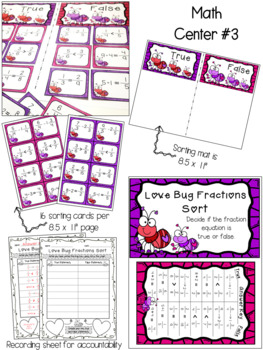# Valentine's Day Fractions Math Centers and Activities

Rated 4.87 out of 5, based on 15 reviews
15 Ratings;
3rd - 5th, Homeschool
Subjects
Resource Type
Standards
Formats Included
• PDF
Pages
30 pages
Report this resource to TPT

#### What educators are saying

These were a little bit of work to print and set up, but it was worth it. My students loved the games and the Valentine's Day theme was perfect.

### Description

ENGAGE your students while they practice different fraction concepts with these 5 math centers. Your students will be begging to do these Math centers during Valentine’s Day and beyond. Easy to set up and eye-catching, to please both the students and you, the teacher!

Activities for students to complete independently and/or with a partner. Ideal for students in Grades 3-5. Set up tips and ink saving options!

Included are 5 Math centers and games that cover the following concepts:

★ Improper fractions

★ Mixed numbers

★ Equivalent fractions

★ Dividing fractions by whole numbers

★ Comparing fractions

Please see the PREVIEW FILE to see exactly what you will be getting with this purchase!

MORE VALENTINE’S DAY MATH CENTERS HERE!

Check out these engaging multiplication activities !

---------------------------------------------------------------------------------------

This resource is not to be shared with colleagues without purchasing the proper number of licenses. This resource may not be uploaded to the internet in any form including classroom/personal websites or shared network drives.

Created by TchrBrowne / Terri’s Teaching Treasures

Total Pages
30 pages
Included
Teaching Duration
N/A
Report this resource to TPT
Reported resources will be reviewed by our team. Report this resource to let us know if this resource violates TPT’s content guidelines.

### Standards

to see state-specific standards (only available in the US).
Recognize and generate simple equivalent fractions, (e.g., 1/2 = 2/4, 4/6 = 2/3). Explain why the fractions are equivalent, e.g., by using a visual fraction model.
Express whole numbers as fractions, and recognize fractions that are equivalent to whole numbers. Examples: Express 3 in the form 3 = 3/1; recognize that 6/1 = 6; locate 4/4 and 1 at the same point of a number line diagram.
Compare two fractions with different numerators and different denominators, e.g., by creating common denominators or numerators, or by comparing to a benchmark fraction such as 1/2. Recognize that comparisons are valid only when the two fractions refer to the same whole. Record the results of comparisons with symbols >, =, or <, and justify the conclusions, e.g., by using a visual fraction model.
Interpret a fraction as division of the numerator by the denominator (𝘢/𝘣 = 𝘢 ÷ 𝘣). Solve word problems involving division of whole numbers leading to answers in the form of fractions or mixed numbers, e.g., by using visual fraction models or equations to represent the problem. For example, interpret 3/4 as the result of dividing 3 by 4, noting that 3/4 multiplied by 4 equals 3, and that when 3 wholes are shared equally among 4 people each person has a share of size 3/4. If 9 people want to share a 50-pound sack of rice equally by weight, how many pounds of rice should each person get? Between what two whole numbers does your answer lie?
Interpret division of a unit fraction by a non-zero whole number, and compute such quotients. For example, create a story context for (1/3) ÷ 4, and use a visual fraction model to show the quotient. Use the relationship between multiplication and division to explain that (1/3) ÷ 4 = 1/12 because (1/12) × 4 = 1/3.